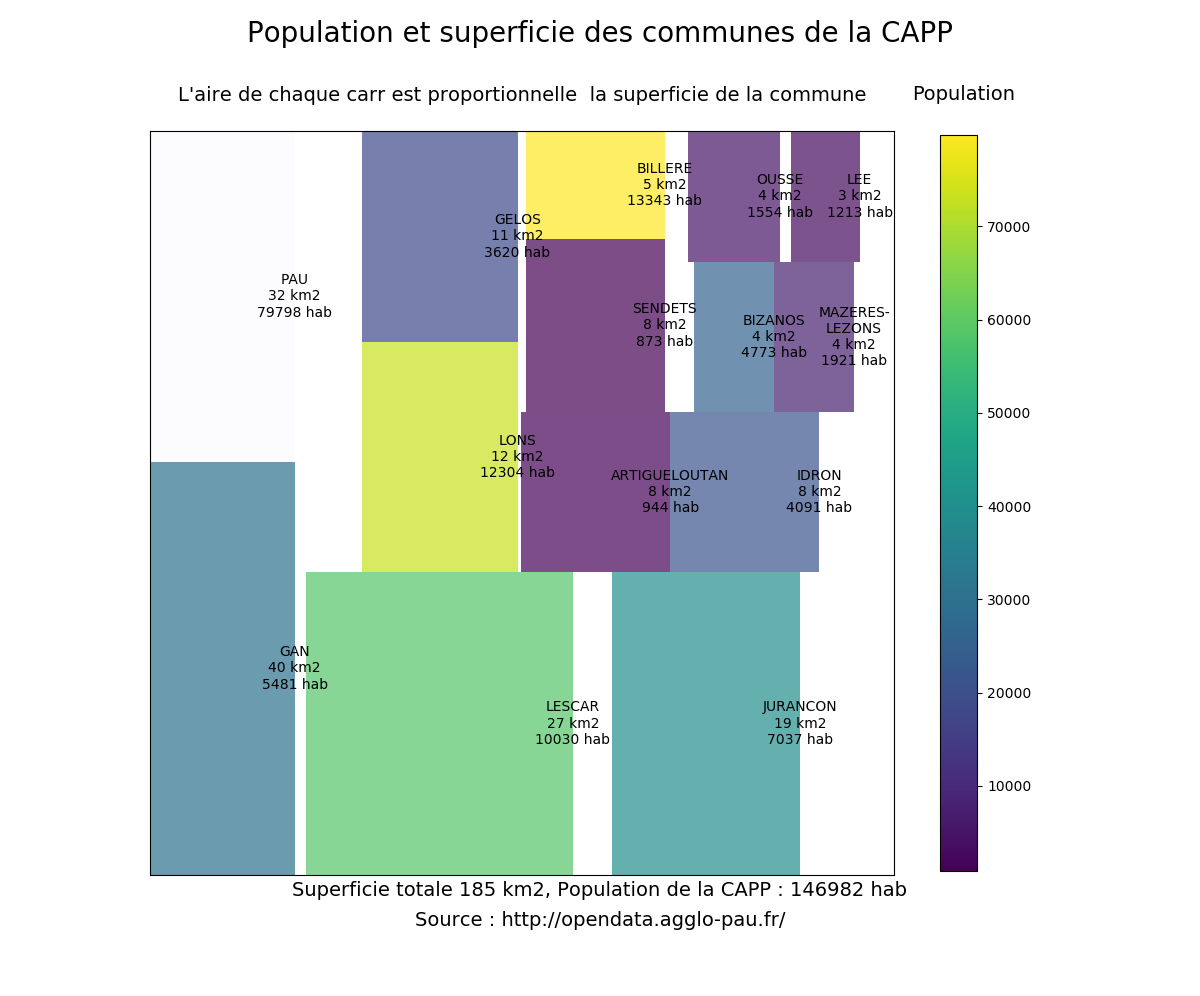Instantly share code, notes, and snippets.

#gVallverdu/capp_treemaps.py Last active Sep 5, 2019

Treemaps with python and matplotlib
 import matplotlib import matplotlib.pyplot as plt import pandas as pd import squarify # qualtities plotted # squarre area is the town surface area (superf) # color scale is the town population in 2011 (p11_pop) # read data from csv file # data from CAPP opendata http://opendata.agglo-pau.fr/index.php/fiche?idQ=27 df = pd.read_csv("Evolution_et_structure_de_la_population/Evolution_structure_population.csv", sep=";") df = df.set_index("libgeo") df = df[["superf", "p11_pop"]] df2 = df.sort_values(by="superf", ascending=False) # treemap parameters x = 0. y = 0. width = 100. height = 100. cmap = matplotlib.cm.viridis # color scale on the population # min and max values without Pau mini, maxi = df2.drop("PAU").p11_pop.min(), df2.drop("PAU").p11_pop.max() norm = matplotlib.colors.Normalize(vmin=mini, vmax=maxi) colors = [cmap(norm(value)) for value in df2.p11_pop] colors = "#FBFCFE" # labels for squares labels = ["%s\n%d km2\n%d hab" % (label) for label in zip(df2.index, df2.superf, df2.p11_pop)] labels = "MAZERES-\nLEZONS\n%d km2\n%d hab" % (df2["superf"]["MAZERES-LEZONS"], df2["p11_pop"]["MAZERES-LEZONS"]) # make plot fig = plt.figure(figsize=(12, 10)) fig.suptitle("Population et superficie des communes de la CAPP", fontsize=20) ax = fig.add_subplot(111, aspect="equal") ax = squarify.plot(df2.superf, color=colors, label=labels, ax=ax, alpha=.7) ax.set_xticks([]) ax.set_yticks([]) ax.set_title("L'aire de chaque carré est proportionnelle à la superficie de la commune\n", fontsize=14) # color bar # create dummy invisible image with a color map img = plt.imshow([df2.p11_pop], cmap=cmap) img.set_visible(False) fig.colorbar(img, orientation="vertical", shrink=.96) fig.text(.76, .9, "Population", fontsize=14) fig.text(.5, 0.1, "Superficie totale %d km2, Population de la CAPP : %d hab" % (df2.superf.sum(), df2.p11_pop.sum()), fontsize=14, ha="center") fig.text(.5, 0.07, "Source : http://opendata.agglo-pau.fr/", fontsize=14, ha="center") plt.show()
Owner Author

### scienceML commented Jan 17, 2017

 Hi there, could you tell what version of python, matplotlib did you use? It does not render like you have on your webpage.

### Michael-E-Rose commented Feb 22, 2017

 Without knowledge of how the data looks like this example is pretty useless.

### hiramf commented Jun 9, 2017

 @Michael-E-Rose I disagree, I've been looking for way to plot treemaps and I find it very helpful. You can look at the data yourself here: Evolution et structure de la population de 1968 à 2012 sur la CAPP

### smxzehvb commented Aug 22, 2017

 You probably want to also scale the colorbar without PAU. Line 46 should probably be: img = plt.imshow([df2.drop("PAU").p11_pop], cmap=cmap)

### yngstr commented Sep 28, 2017 • edited

 I've tried replicating your example with the data from the link, code runs fine but the treemap has overlapping rectangles and labels, and is generally unreadable/not useful. Mind sharing your versions? using python 2.7 and matplotlib 2.0.2### natngmk commented Sep 30, 2017

 Hi, this is some thing useful. However, learning from your example, my boxes in the plot doesn't have any border. Did I miss something?

### dannikaaa commented Jan 18, 2019

 Hi how did you get the border?

### taniki commented Jan 25, 2019 • edited

 @dannikaaa `squarify.plot()` allows you to add extra parameters to`matplotlib.pyplot.bar` ex: ``````squarify.plot(..., linewidth=2, edgecolor="#ffffff") ``````

### cameronabrams commented Feb 6, 2019

 In my hands, I need to pass linewidth and edgecolor in the dict bar_kwargs: ` squarify.plot(...., bar_kwargs={'linewidth':2, 'edgecolor':'#000000'})`
to join this conversation on GitHub. Already have an account? Sign in to comment
You can’t perform that action at this time.